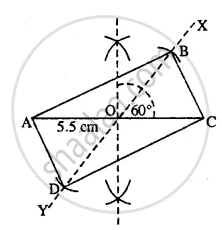# Construct a Rectangle Abcd; If : Each Diagonal is 5.5 Cm and the Angle Between Them is 60°. - Mathematics

Sum

Construct a rectangle ABCD; if :

each diagonal is 5.5 cm and the angle between them is 60°.

#### SolutionSteps :

1. Draw AC = 5.5 cm.
2. Bisect AC at O.
3. At O, draw ∠XOC = 60° and produce XO to Y.
4. Cut OB = OA and OD = OA (half the diagonal AC).
5. Join AB, BC, AD, and CD.
Thus ABCD is the required rectangle.
Concept: Construction of a Rectangle When Its Length and Breadth Are Given.
Is there an error in this question or solution?

#### APPEARS IN

Selina Concise Mathematics Class 8 ICSE
Chapter 18 Constructions
Exercise 18 (D) | Q 3.6 | Page 211